# (The following information applies to Questions 3 and 4)​ You observe the following information in a market where the CAPM holds: beta Expected return Annual standard deviation Stock A 1.5 15.0% 0.25 Stock B 1.2 13.2% 0.30 The correlation co

(The following information applies to Questions 3 and 4)

You observe the following information in a market where the CAPM holds:

 beta Expected return Annual standard deviation Stock A 1.5 15.0% 0.25 Stock B 1.2 13.2% 0.30

The correlation coefficient between stock A and the market is 60%.

Question 3:

Compute the expected return on the market portfolio.

Question 4:

What is the expected return of a portfolio that is split (perhaps unevenly) between the risk-free asset and the market, if this portfolio has a standard deviation of 0.07?

This Homework Help Question: "(The following information applies to Questions 3 and 4)​ You observe the following information in a market where the CAPM holds: beta Expected return Annual standard deviation Stock A 1.5 15.0% 0.25 Stock B 1.2 13.2% 0.30 The correlation co" No answers yet.

We need 10 more requests to produce the answer to this homework help question. Share with your friends to get the answer faster!

0 /10 have requested the answer to this homework help question.

Once 10 people have made a request, the answer to this question will be available in 1-2 days.
All students who have requested the answer will be notified once they are available.
##### Add Answer to: (The following information applies to Questions 3 and 4)​ You observe the following information in a market where the CAPM holds: beta Expected return Annual standard deviation Stock A 1.5 15.0% 0.25 Stock B 1.2 13.2% 0.30 The correlation co
Similar Homework Help Questions
• ### Stock X has an expected return of 15%, standard deviation of 20%, beta of 0.8. Stock...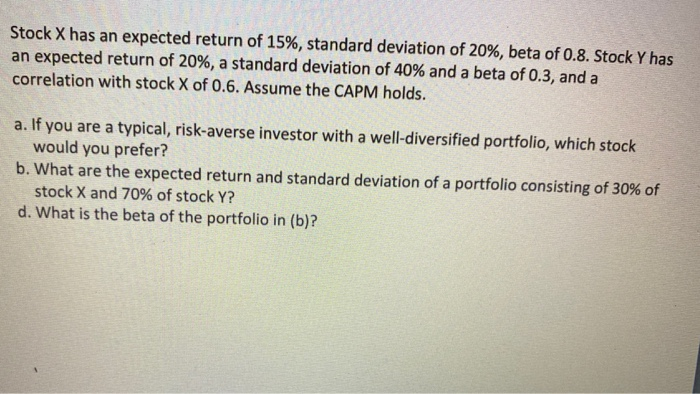Stock X has an expected return of 15%, standard deviation of 20%, beta of 0.8. Stock Y has an expected return of 20%, a standard deviation of 40% and a beta of 0.3, and a correlation with stock X of 0.6. Assume the CAPM holds. a. If you are a typical, risk-averse investor with a well-diversified portfolio, which stock would you prefer? b. What are the expected return and standard deviation of a portfolio consisting of 30% of stock X...

• ### Assume that the assumptions of the CAPM hold. The expected return and the standard deviation of...

Assume that the assumptions of the CAPM hold. The expected return and the standard deviation of the market portfolio are 7% and 14%, respectively. There are two individual stocks A and B: Mean Return A: 4% Standard Deviation A: 18% Mean Return B: 12% Standard Deviation B: 36% Stock A has a correlation of 0.2 with the market portfolio. A.What is the beta of stock A? B.What is the risk free rate? C.What is the beta of a portfolio with...

• ### Stock A has an expected return of 11 percent, a beta of 0.9, and a standard deviation of 15 perce...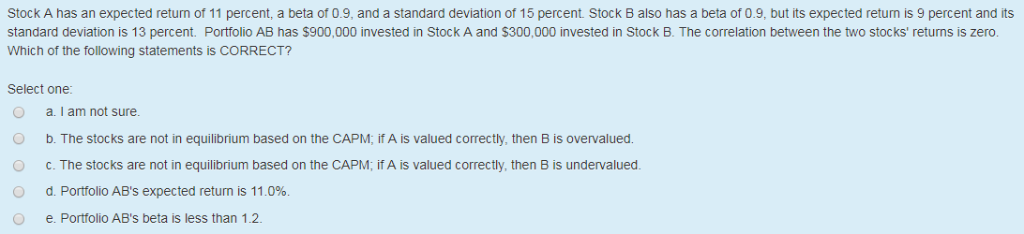Stock A has an expected return of 11 percent, a beta of 0.9, and a standard deviation of 15 percent Stock B also has a beta of 0.9, but its expected returm is 9 percent and its standard deviation is 13 percent. Portfolio AB has \$900,000 invested in Stock A and \$300,000 invested in Stock B. The correlation between the two stocks' returns is zero. Which of the following statements is CORRECT? Select one O a.I am not sure b....

• ### EXTRA RISK PROBLEMS Stock A Stock B Expected Return 10% 1 Standard Deviation Beta Correlation coefficient with the...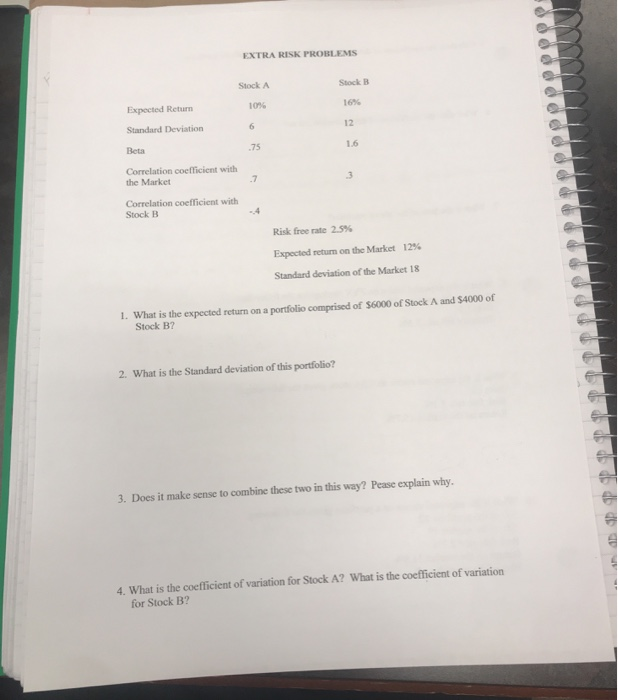EXTRA RISK PROBLEMS Stock A Stock B Expected Return 10% 1 Standard Deviation Beta Correlation coefficient with the Market Correlation coefficient with Stock B Risk free rate 25% Expected return on the Market 12% Standard deviation of the Market 18 1. What is the expected return on a portfolio comprised of \$6000 of Stock A and S4000 of Stock B? 2. What is the Standard deviation of this portfolio? 3. Does it make sense to combine these two in this...

• ### EXTRA RISK PROBLEMS Stock A Stock B Expected Return 10% 16% Standard Deviation Correlation coefficient with...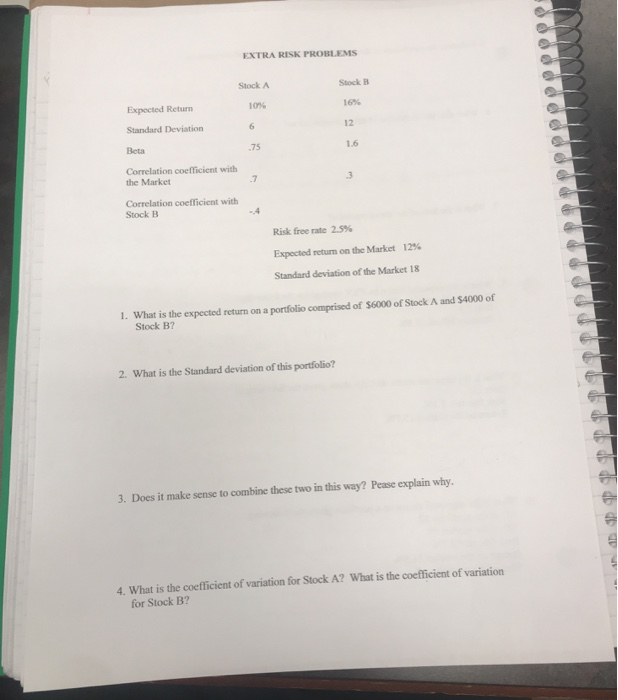EXTRA RISK PROBLEMS Stock A Stock B Expected Return 10% 16% Standard Deviation Correlation coefficient with the Market Correlation coefficient with Stock B Risk free rate 25% Expected return on the Market 12% Standard deviation of the Market 18 1. What is the expected return on a portfolio comprised of \$6000 of Stock A and \$4000 of Stock B? 2. What is the Standard deviation of this portfolio? 3. Does it make sense to combine these two in this way?...

• ### 2. Consider the information in Table1. Table 1 Standard Deviation of Stock Stock Correlation with Market...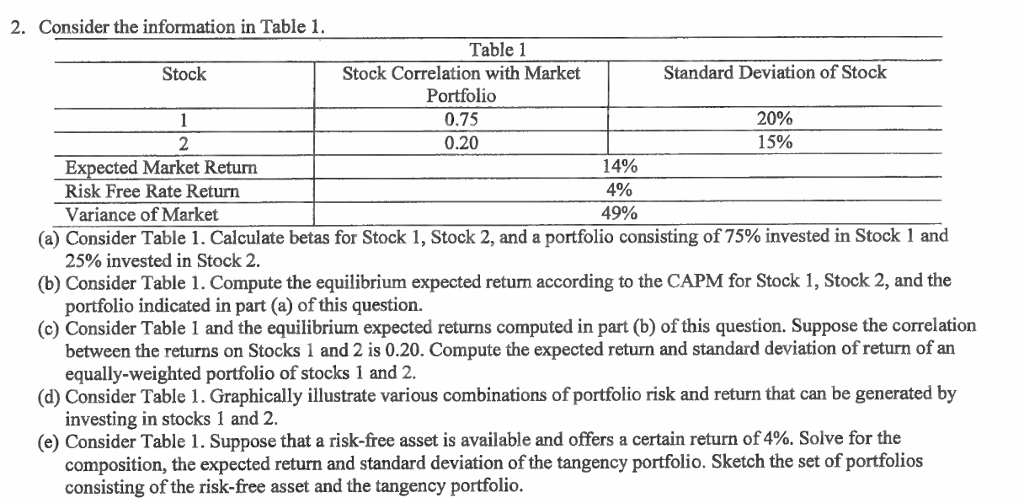2. Consider the information in Table1. Table 1 Standard Deviation of Stock Stock Correlation with Market Portfolio 0.75 0.20 Stock 20% 15% 14% 0% 49% ected Market Return Risk Free Rate Return (a) Consider Table 1 . Calculate betas for Stock 1, Stock 2, and a portfolio consisting of 75% invested in Stock 1 and (b) Consider Table 1. Compute the equilibrium expected return according to the CAPM for Stock 1, Stock 2, and the (c) Consider Table 1 and...

• ### 2. Company A's stock has a beta of BA 1.5, and Company B's stock has a beta of βΒ-2.5. Expected r...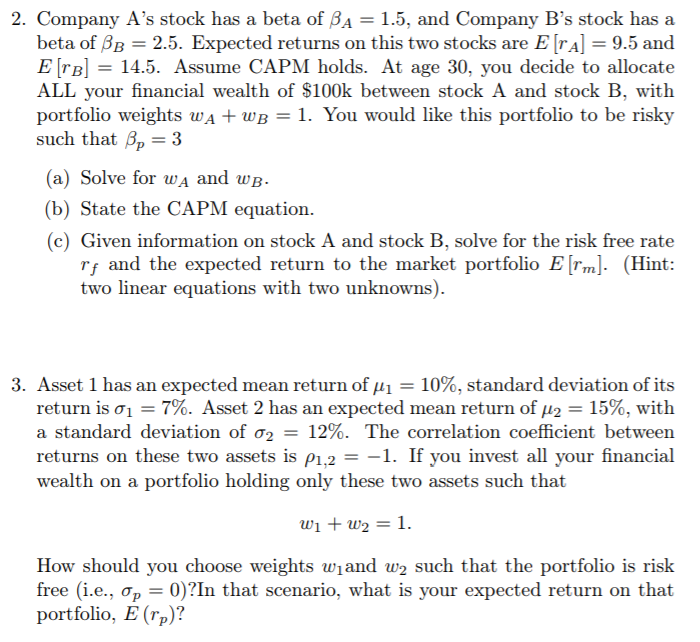2. Company A's stock has a beta of BA 1.5, and Company B's stock has a beta of βΒ-2.5. Expected returns on this two stocks are E [rA]-9.5 and E rB 14.5. Assume CAPM holds. At age 30, you decide to allocate ALL your financial wealth of \$100k between stock A and stock B, with portfolio weights wA + wB1. You would like this portfolio to be risky such that Bp- 3 (a) Solve for wA and wB- (b) State...

• ### Asset A has a CAPM beta of 1.5. The covariance between asset A and asset B is 0.13. If the risk-free rate is 0.05, the expected market risk premium is 0.07, and the market risk premium has a standard...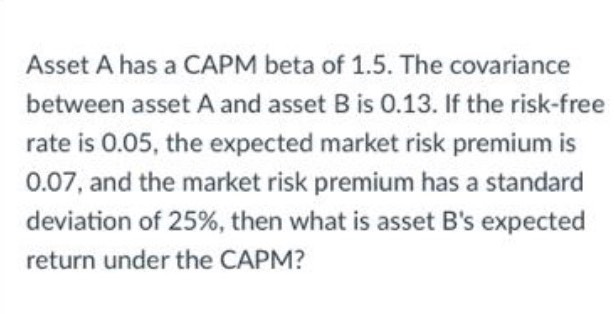Asset A has a CAPM beta of 1.5. The covariance between asset A and asset B is 0.13. If the risk-free rate is 0.05, the expected market risk premium is 0.07, and the market risk premium has a standard deviation of 25%, then what is asset B's expected return under the CAPM? Asset A has a CAPM beta of 1.5. The covariance between asset A and asset B is 0.13. If the risk-free rate is 0.05, the expected market risk...

• ### Given the following: Stock A Expected return= 0.28, standard deviation = 0.40 Stock B Expected return=...

Given the following: Stock A Expected return= 0.28, standard deviation = 0.40 Stock B Expected return= 0.16, standard deviation = 0.25 If stock A and stock B have a positive correlation of 0.48, which portfolio represent the minimum variance portfolio? Weight of Stock A in the minimum variance portfolio: _____ Weight of Stock B in the minimum variance portfolio: _____ The expected return and standard deviation of this minimum variance portfolio: ______ and ________ show all formulas and calculations please

• ### The following are estimates for two stocks. Firm-Specific Standard Deviation Stock A B Expected Return 108...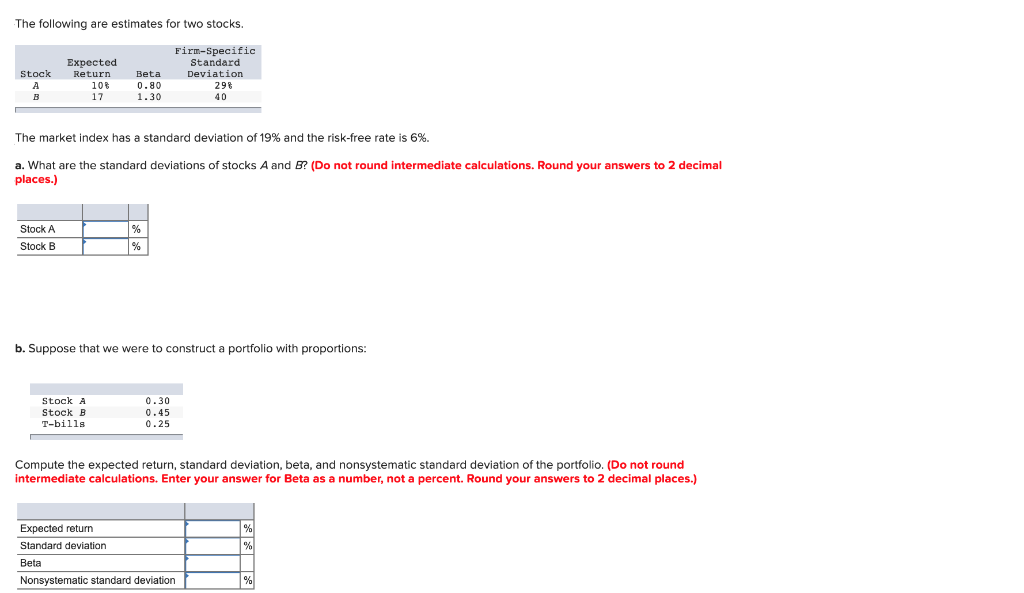The following are estimates for two stocks. Firm-Specific Standard Deviation Stock A B Expected Return 108 17 Beta 0.80 1.30 298 40 The market index has a standard deviation of 19% and the risk-free rate is 6%. a. What are the standard deviations of stocks A and B? (Do not round Intermediate calculations. Round your answers to 2 decimal places.) Stock A Stock B b. Suppose that we were to construct a portfolio with proportions: Stock A Stock B T-bills...

Free Homework App